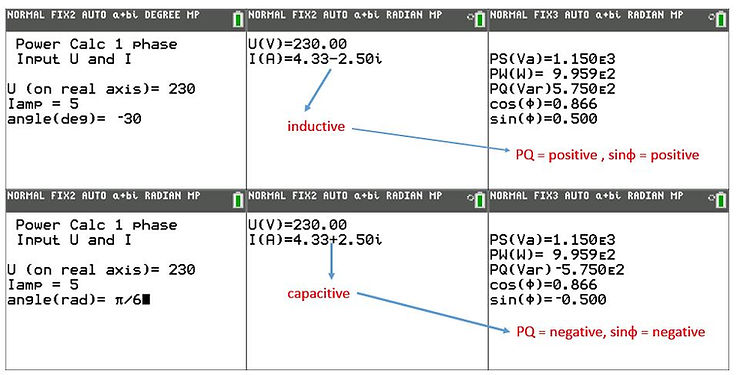naver-site-verification: naver47238a1b6bfbb19a2fd4b619734fa9a6.html
top of page

# Power, reactive and apparent power and power factor calculations  (for sinusoidal Voltage and Current)

## Explanation of the power calculator programs for Ti-84: ONEPHASE.8XP

### Definitions

The calculation of power, power factor, reactive power and current is a very important subject in Electrical Engineering.

4 Different inputs in this Ti program are possible.

Definitions according to electrical network theory for sinusoidal signals.

Formulas: Pc=U.I *= complex power, I*= conjugate of I, definition conjugate:  (a+bi)*=(a-bi)

abs(Pc)=apparent power (VA)=Pa,

Re(Pc)= real power (Watt)=Ptrue,

Im(Pc)=reactive power (Var)= Pr

cos(ϕ) or power factor (Pf)= (real power)/(apparent power)=Ptrue/Pa

More basic information is available on: